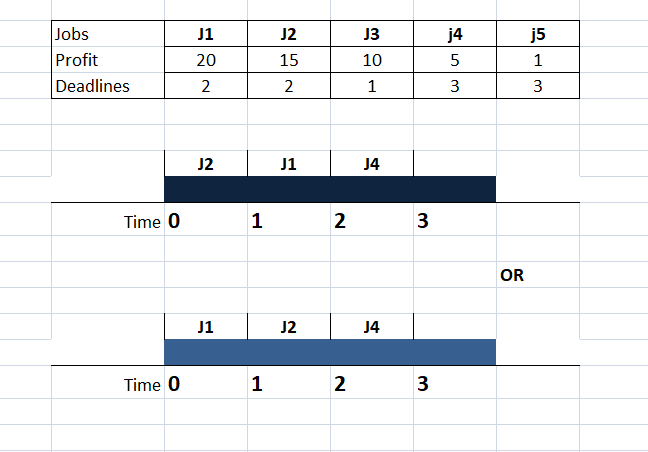# Job Sequencing Problem using Greedy method in Java

In this article, we will see the concepts of Job sequencing Problem with DeadLine in Java using Greedy Algorithm.
In this problem, We want set of those Jobs which can be completed within their deadlines, Such that their profit is maximized.

### Concept of Job Sequencing Problem in Java

Job-Sequence problem consists of certain finite jobs associated with their deadline and profits.
Our goal is to earn maximum profit.
We will assume that each job takes 1 unit time to traverse, So the minimum deadline for each job is 1.
We will earn profit from a particular job only when it is completed before or on time.Final hr(unit):3

Step 1:Look for the maximum profit (J1:20) and it is ready to wait for 2 units of time. 0->1->2(put J1 in place of 1->2),Insertion is done from back.
Step 2:Look for the second maximum profit(J2:15) and it is also ready to wait for 2 units of time.0->1->2(Put J2 in place of 0->1)
J3 can’t be adjusted because it only can wait for the time (0->1), and 0-> is already filled with J1.
Step 3:Repeat step 1 &2

Final Sequence  :  J2—> J1—>j4
or, J1—>J2—>J4
Total Profit  :  20 + 15 + 5 = 40

#### Constraints taken while writing the algorithm

• Each Job takes 1 unit of time.
• Arrange profit in decending order.
• Let’s suppose, each Job need 1 hr for completion & J1 is ready to wait for 1 hrs, J4 is ready to wait for 3 hrs.
• Nobody is ready to wait beyong 3 hrs.
• J4 job is done in 1 hrs, but he is ready to wait for 3 hrs, But we have to look for the for the profit(Max. Profit comes first).
```import java.util.*;
public class job
{
public static void main(String args[])
{
Scanner sc=new Scanner(System.in);
System.out.println("Enter the number of Jobs");
int n=sc.nextInt();
String a[]=new String[n];
int b[]=new int[n];
int c[]=new int[n];
for(int i=0;i<n;i++)
{
System.out.println("Enter the Jobs");
a[i]=sc.next();
System.out.println("Enter the Profit");
b[i]=sc.nextInt();
c[i]=sc.nextInt();
}
System.out.println("--Arranged Order--");
System.out.print("Jobs:    ");
for(int i=0;i<n;i++)
{
System.out.print(a[i]+" ");
}
System.out.println();
System.out.print("Profit:  ");
for(int i=0;i<n;i++)
{
System.out.print(b[i]+" ");
}
System.out.println();
for(int i=0;i<n;i++)
{
System.out.print(c[i]+" ");
}
for(int i=0;i<n-1;i++)
{
for(int j=i+1;j<n;j++)
{
if(b[i]<b[j])
{
int temp=b[i];
b[i]=b[j];
b[j]=temp;

temp=c[i];
c[i]=c[j];
c[j]=temp;

String temp1=a[i];
a[i]=a[j];
a[j]=temp1;
}
}
}
System.out.println();
System.out.println("--Sorted Order--");
System.out.print("Jobs:    ");
for(int i=0;i<n;i++)
{
System.out.print(a[i]+" ");
}
System.out.println();
System.out.print("Profit:  ");
for(int i=0;i<n;i++)
{
System.out.print(b[i]+" ");
}
System.out.println();
for(int i=0;i<n;i++)
{
System.out.print(c[i]+" ");
}
System.out.println();
int max=c;
for(int i=0;i<n;i++)
{
if(c[i]>max)
{
max=c[i];
}
}
String x[]=new String[max];
int xx[]=new int[max];
int profit=0;
for(int i=0;i<n;i++)
{
int pp=c[i];
pp=pp-1;
if(x[pp]==null )
{
x[pp]=a[i];
profit+=b[i];
}
else
{
while(pp!=-1)
{
if(x[pp]==null)
{
x[pp]=a[i];
profit+=b[i];
break;
}
pp=pp-1;
}
}
}
for(int i=0;i<max;i++)
{
System.out.print("-->"+x[i]);
}
System.out.println();
System.out.print("Profit Earned"+profit);
}
}
```
##### Output:
```Enter the number of Jobs
7
Enter the Jobs
J1
Enter the Profit
35
3
Enter the Jobs
J2
Enter the Profit
30
4
Enter the Jobs
J3
Enter the Profit
25
4
Enter the Jobs
J4
Enter the Profit
20
2
Enter the Jobs
J5
Enter the Profit
15
3
Enter the Jobs
J6
Enter the Profit
12
1
Enter the Jobs
J7
Enter the Profit
5